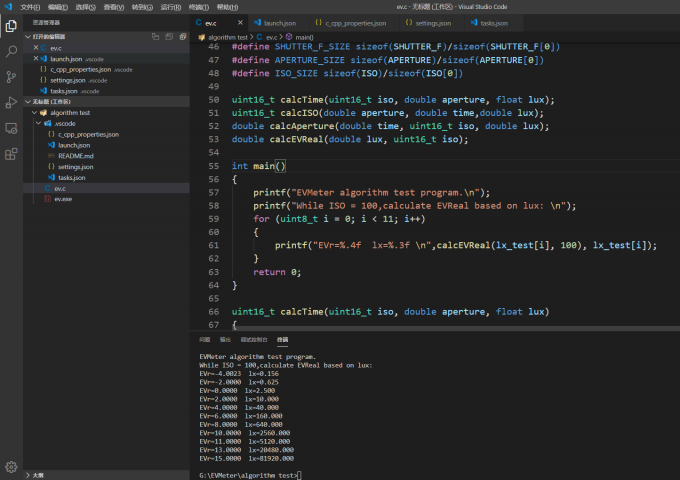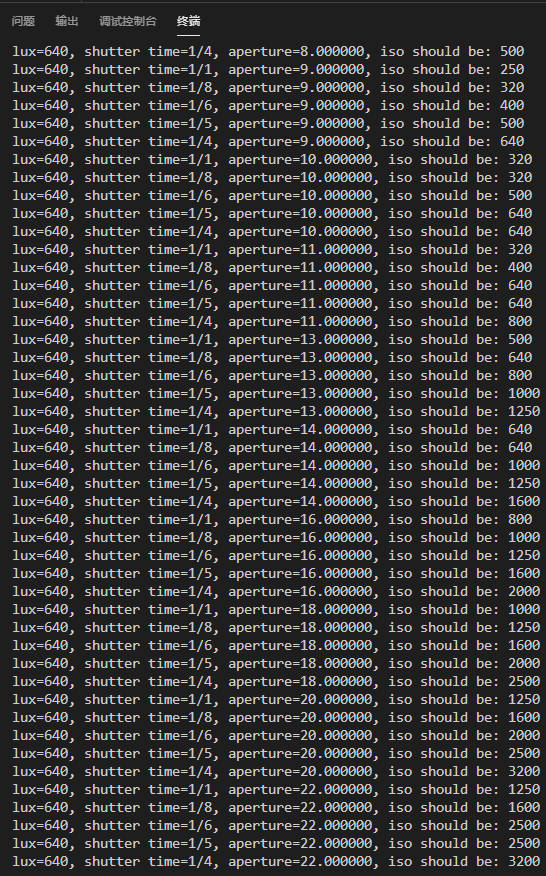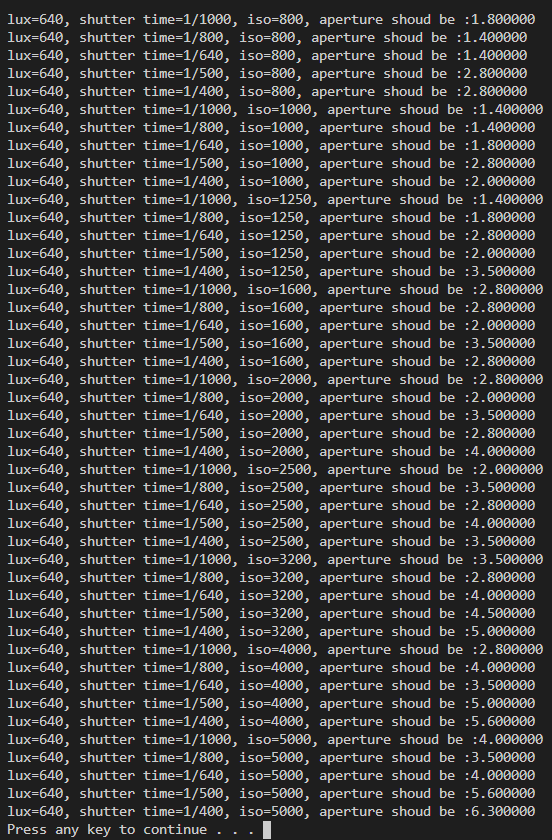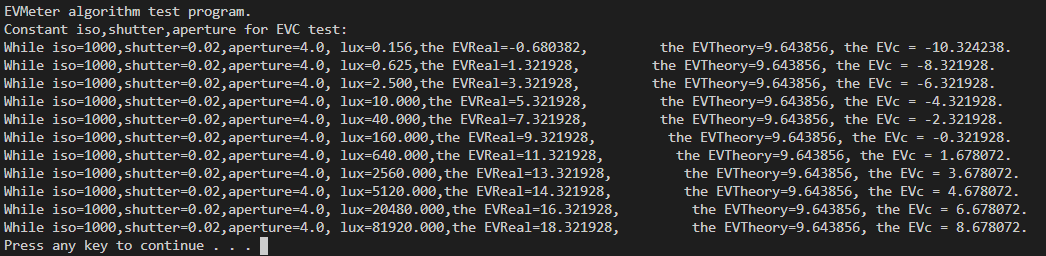# EV-Exposure Value

## Define

$E_v = \log_2 {N^2 \over t}$

## EV & Light(Lux)

${N^2 \over t} = {LS \over K}$

• N是相对光圈(F值)
• t是曝光时间,单位秒(s)
• L是场景平均辉度(就是平均亮度)
• S是ISO指数
• K是反射式测光表校正常数

$E_v = \log_2{LS \over K}$

${N^2 \over t} = {ES \over C}$

• E是照度
• C是入射式测光表校正常数
当然也有:

$E_v = log_2{ES \over C}$

# 用EV作为照度与亮度的量度

$L = 2^{EV-3}$

$EV = log_2{N^2 \over t}$

${N^2 \over t} = {LS \over K}$

$\Longrightarrow L = 2^{EV}*{K \over S}$

$while K=12.5,S=100, \Longrightarrow L = 2^{EV}*0.125=2^{EV-3}$

$E = 2.5*2^{EV}$

$EV = log_2{N^2 \over t}$

${N^2 \over t} = {ES \over C}$

$\Longrightarrow E = 2^{EV}*{C \over S}$

$while C=250,S=100, \Longrightarrow L = 2.5*2^{EV}$

### Table

EV 亮度(cd/m^2) 照度(lx) w分割行w EV 亮度(cd/m^2) 照度(lx)
-4 0.008 0.156 www 7 16 320
-3 0.016 0.313 www 8 32 640
-2 0.031 0.625 www 9 64 1280
-1 0.063 1.25 www 10 128 2560
0 0.125 2.5 www 11 256 5120
1 0.250 5 www 12 512 10240
2 0.5 10 www 13 1024 20480
3 1 20 www 14 2048 40960
4 2 40 www 15 4096 81920
5 4 80 www 16 8192 163840
6 8 160 www of! of! overflow!

# 对于不同的ISO(这次是感光度了)的修正

$EV_S = EV_{100} + log_2{S \over 100}.$

$EV_{400} = EV_{100} + log_2{400 \over 100} = EV_{100} + 2.$

$EV_{50} = EV_{100} + log_2{50 \over 100} = EV_{100} - 1.$

# 再进一步!

1. 指定ISO、光圈、快门
2. 看一下曝光补偿的提示，是欠曝了还是过曝了,适当调整拍摄参数
3. Shoot!

• 输入3个参数
• 根据传感器获取的光照度和指定的ISO来计算被拍摄场景的EV值，记作EVr(Real)
• 根据3个参数算出EV值，记作EVt(theoretical)
• 两者做差，得到曝光补偿

• 输入2个参数
• 根据公式直接定出第三个参数,此时的EVr与EVt理论上来说完全相等
• 但是由于参数刻度非线性,不可能完全相等。

$t = {N^2 \over S*E} * C$

$S = { { N^2 * E } \over t} * {1 \over C}$

$N = \sqrt {S*E*t \over C}$

• t-快门时间,单位秒(s)
• N-光圈F值,典型的如f2.8、f4、f5.6…
• S-ISO值,典型的如100、200、320、400、800、1600…
• E-入射式测光传感器得到的照度值,单位lux(勒克斯),由传感器给出
• C-平面型传感器测量照度时的校正常数,默认250,需要根据硬件结构等不定因素适当调整

# Codeworks## 根据ISO/Aperture/Lux计算时间

$t = {N^2 \over S*E} * C$

## Test!# 下一站！

## Reference

------ 本文结束，感觉您的阅读 ------